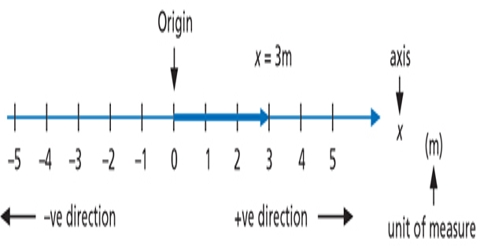# One Dimensional Reference Frame

One dimensional reference frame:

Suppose a particle is moving along straight line OX. The positions of the particle at different times are determined with respect to a fixed point. This fixed point with respect to which the position of the particle is determined is called reference point or index point. In the figure O has been taken as the reference point. The straight line OX is called X-axis. One dimensional reference frame has been formed with the reference point O and X-axis. By the help of this frame reference any particle at any time can be determined. [Fig]Suppose the particle is at position A at a particular tune. The distance of the particle from the point O at that time = OA = x. If the particle is at rest, then z will have only one value, but if it is in motion then x will have different values. Here x is called co-ordinate. The position of the particle is determined by only one co-ordinate, so the particle is located at one dimensional space. If position of different particles of a body is determined by one co-ordinate, so the body is called one dimensional body.The position of a freely falling body at different times is different and can be expressed by a single co-ordinate. The point from where the body starts falling is called reference point and the path of its motion will be considered X-axis.

Examples: A long thin rod. a long thin thread. a hanging thread etc. may be taken as one dimensional objects.